## Answers to exercises

##### Exercise 1

Let $$A(x_1,y_1)$$ and $$B(x_2,y_2)$$ be two points. Assume that $$P(x,y)$$ is on the interval $$AB$$ with

$AP:PB = a:b = \tfrac{a}{b}:1.$

So $$P$$ divides $$AB$$ in the ratio $$\dfrac{a}{b}:1$$. Thus

$x = \dfrac{x_1+\dfrac{a}{b}x_2}{1+\dfrac{a}{b}} = \dfrac{bx_1+ax_2}{b+a}$

and, similarly,

$y = \dfrac{by_1+ay_2}{b+a}.$
##### Exercise 2

Without loss of generality, assume $$PB = 1$$. Then

\begin{align*} AC &= AP\,\cos\theta\\ x-x_1 &= k\,\dfrac{x_2-x_1}{k+1}\\ (x-x_1)(k+1) &= kx_2-kx_1\\ x &= \dfrac{x_1+kx_2}{k+1}. \end{align*}

From the same diagram, we have $$CP = AP\,\sin\theta$$. Proceed in a similar way to find $$y$$.

##### Exercise 3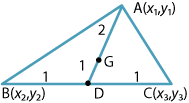Detailed description

The midpoint $$D$$ of $$BC$$ is

$\Bigl(\dfrac{x_2+x_3}{2},\dfrac{y_2+y_3}{2}\Bigr).$

The point $$G$$ that divides the median $$AD$$ in the ratio $$2:1$$ is

$\Biggl(\dfrac{1}{3}\Bigl(x_1 + \dfrac{2(x_2+x_3)}{2}\Bigr), \dfrac{1}{3}\Bigl(y_1 + \dfrac{2(y_2+y_3)}{2}\Bigr)\Biggr) = \Biggl(\dfrac{x_1+x_2+x_3}{3}, \dfrac{y_1+y_2+y_3}{3}\Biggr).$

This is symmetric in $$x_1,x_2,x_3$$ and in $$y_1,y_2,y_3$$. So $$G$$ lies on the other medians $$BE$$ and $$CF$$, and the medians are concurrent.

##### Exercise 4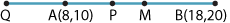$$M$$ is $$(13,15)$$.

$$P$$ is $$\Bigl(\dfrac{3\times 8+2\times 18}{3+2}, \dfrac{3\times 10+2\times 20}{3+2}\Bigr) = (12,14)$$.

$$Q$$ is $$\Bigl(\dfrac{3\times 8-2\times 18}{3-2}, \dfrac{3\times 10-2\times 20}{3-2}\Bigr) = (-12,-10)$$.

Thus

\begin{align*} MB^2 &= 5^2+5^2=50,\\ MP^2 &= 1^2+1^2=2,\\ MQ^2 &= 25^2+25^2=2\times 25^2. \end{align*}

Hence

$MP \cdot MQ = \bigl(2\times 2\times 25^2\bigr)^{\frac{1}{2}} = 50 = MB^2.$
##### Exercise 5
1. $$\text{Gradient} = \dfrac{\dfrac{c}{q}-\dfrac{c}{p}}{cq-cp} = \dfrac{p-q}{pq(q-p)} = -\dfrac{1}{pq}$$
2. $$\text{Gradient} = \dfrac{2aq-2ap}{aq^2-ap^2} = \dfrac{2(q-p)}{(q-p)(q+p)} = \dfrac{2}{p+q}$$
3. $$\text{Gradient} = \dfrac{b\sin\phi-b\sin\theta}{a\cos\phi-a\cos\theta} = \dfrac{2b\,\cos\bigl(\dfrac{\phi+\theta}{2}\bigr)\,\sin\bigl(\dfrac{\phi-\theta}{2}\bigr)} {2a\,\sin\bigl(\dfrac{\phi+\theta}{2}\bigr)\,\sin\bigl(\dfrac{\theta-\phi}{2}\bigr)} = -\dfrac{b}{a}\cot\Bigl(\dfrac{\phi+\theta}{2}\Bigr)$$
##### Exercise 6
1. The lines $$4x+3y-6=0$$ and $$x-2y-7=0$$ meet where $$11y+22=0$$, so $$y=-2$$ and $$x=3$$. The line through $$(3,-2)$$ parallel to the $$x$$-axis is $$y=-2$$.
2. The lines $$3x+2y=2$$ and $$4x+3y=7$$ meet where $$y=13$$ and $$x=-8$$. So the gradient is $$\dfrac{13-3}{-8-2}=-1$$.
##### Exercise 7

The angle sum formulas give

\begin{align*} \sin(\theta+90^\circ) &= \sin\theta\,\cos 90^\circ + \cos\theta\,\sin 90^\circ = \cos\theta\\ \cos(\theta+90^\circ) &= \cos\theta\,\cos 90^\circ - \sin\theta\,\sin 90^\circ = -\sin\theta. \end{align*}

Thus

$\tan(90^\circ+\theta) = \dfrac{\cos\theta}{-\sin\theta} = -\dfrac{1}{\tan\theta}.$

Suppose $$y=mx$$ is perpendicular to $$y=m_1x$$. Then $$m=\tan\theta$$ and $$m_1=\tan(90^\circ +\theta)$$. So

$m_1=-\dfrac{1}{m}$

and therefore $$mm_1=-1$$.

Conversely, suppose $$mm_1=-1$$, where $$m =\tan\theta$$. Then

$m_1 = -\dfrac{1}{\tan\theta} = \tan(90^\circ + \theta).$

So $$y=mx$$ is perpendicular to $$y=m_1x$$.

##### Exercise 8
1. All lines parallel to $$ax+by+c = 0$$ have equation $$ax+by+d = 0$$, for some $$d$$. If $$P(x_1,y_1)$$ lies on the line, then $$ax_1+by_1+d = 0$$. So the equation is $$ax+by = ax_1+by_1$$.
2. All lines perpendicular to $$l$$ have equation $$bx-ay+d = 0$$, for some $$d$$. If $$P(x_1,y_1)$$ lies on the line, then $$bx_1-ay_1+d = 0$$. So the equation is $$bx-ay = bx_1-ay_1$$.
##### Exercise 9
1. The perpendicular distance of $$(1,2)$$ from the line $$x+2y-10=0$$ is
$\dfrac{|1+4-10|}{\sqrt{1^2+2^2}} = \sqrt{5},$ which equals the radius. Therefore the line is tangent to the circle.
2. The perpendicular distance of $$(1,2)$$ from the line $$x+2y-12=0$$ is
$\dfrac{|1+4-12|}{\sqrt{1^2+2^2}} = \dfrac{7}{\sqrt{5}} = \dfrac{7\sqrt{5}}{5},$ which is greater than the radius $$\sqrt{5}$$. Hence the line does not touch the circle.
##### Exercise 10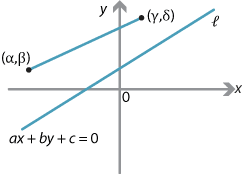Detailed description

Note that a point $$(x_1,y_1)$$ lies on the line $$l$$ if and only if $$ax_1+by_1+c = 0$$.

Suppose that points $$(\alpha,\beta)$$ and $$(\gamma,\delta)$$ are on the same side of $$l$$. Then the line segment from $$(\alpha,\beta)$$ and $$(\gamma,\delta)$$ does not cross $$l$$. So $$ax_1+by_1+c \ne 0$$ for each point $$(x_1,y_1)$$ on the line segment. Thus the expression $$ax_1+by_1+c$$ cannot change sign as the point $$(x_1,y_1)$$ moves along the line segment from $$(\alpha,\beta)$$ to $$(\gamma,\delta)$$. Hence the signs of $$a\alpha+b\beta+c$$ and $$a\gamma+b\delta+c$$ are the same.

Points on different sides of $$l$$ must have different signs, as it is possible to choose $$(x_1,y_1)$$ so that $$ax_1+by_1+c > 0$$, and choose $$(x_2,y_2)$$ so that $$ax_2+by_2+c < 0$$.

##### Exercise 11
\begin{align*} (x+1)^2+(y-6)^2 &= (x-3)^2+(y-4)^2\\ x^2+2x+1+y^2-12y+36 &= x^2-6x+9+y^2-8y+16\\ 2x-12y+37 &= -6x-8y+25\\ 8x-4y+12 &=0\\ 2x-y+3 &= 0 \end{align*}
##### Exercise 12

The area of the triangle is

$\Bigl|\dfrac{1}{2}\bigl(6(5-7)+8(3-5)+2(7-3)\bigr)\Bigr| = \Bigl|\dfrac{-12-16+8}{2}\Bigr| = 10.$
##### Exercise 13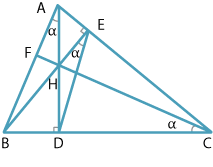Detailed description

Let $$AD$$ be the altitude perpendicular to $$BC$$, let $$BE$$ be the altitude perpendicular to $$AC$$ and let $$AD$$ meet $$BE$$ at $$H$$. Finally let $$CH$$ meet $$AB$$ at $$F$$. We must prove $$FC$$ is perpendicular to $$AB$$.

Join $$DE$$. Let $$\alpha = \angle BCF$$. Then $$ECDH$$ is a cyclic quadrilateral (supplementary opposite angles), so $$\angle DEH=\alpha$$ (angles in the same segment).

Similarly, $$AEBD$$ is a cyclic quadrilateral and $$\angle DAB=\alpha$$.

From $$\triangle ABD$$, $$\angle ABD=180^{\circ} -90^{\circ} -\alpha=90^{\circ} -\alpha$$. Finally, in $$\triangle CBF$$, $$\angle BFC+\alpha+90^{\circ} -\alpha=180^{\circ}$$, so $$\angle BFC=90^{\circ}$$, as required.

##### Exercise 14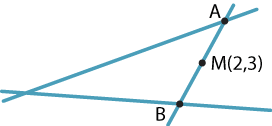Let $$A = (a,b)$$ and $$B = (c,d)$$. The midpoint $$M$$ of $$AB$$ is $$(2,3)= \Bigl(\dfrac{a + c}{2}, \dfrac{b + d}{2}\Bigr)$$, so

\begin{alignat*}{2} a + c &= 4 &\qquad&(1)\\ b + d &= 6. &&(2) \end{alignat*}

Since the point $$A(a,b)$$ lies on the line $$2x + y - 3 = 0$$ and the point $$B(c,d)$$ lies on the line $$3x - 2y + 1 = 0$$, we also have

\begin{alignat*}{2} 2a + b &= 3 &\qquad&(3)\\ 3c - 2d &= -1. &&(4) \end{alignat*}

From (1) and (2), we have $$c = 4 - a$$ and $$d = 6 - b$$. Substituting these into (4) gives

$3a -2b = 1.\qquad (5)$

We can now solve (3) and (5) to obtain $$a = 1$$ and $$b = 1$$.

We have found that $$A$$ is $$(a,b) = (1,1)$$. So the gradient of the line $$l$$ is $$m = \dfrac{1-3}{1-2} = 2$$. Thus $$l$$ has equation $$y - 1 = 2(x - 1)$$ or, equivalently, $$y = 2x - 1$$.